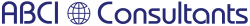﻿ Dictionary - Standard Operators

# Dictionary - Standard Operators

 QMSCAPA Report Builder Features The language used to create this Report Builder allows you to use 'operators' to create and/or compare values stored in your database file(s). Operators are broken into two different groups:   Arithmetic  Addition - Add two variables together to produce a value. The addition sign (+) is used.  Subtraction - Subtraction one variable from another to produce a value. The minus sign (-) is used.  Multiplication - Multiply one variable against another to produce a value. The asterisk symbol (*) is used.  Division - Divide one variable into another to produce a value. The slash character is used (/).  Exponentiation - Raises one variable to the value of a second variable. The carat symbol (^) is used.  Modulus Division - Returns the remainder of one variable divided by another variable. The percentage character (%) is used.   Logical  Choose() - This function is used to perform a logical evaluation and return a value based upon the result. When a condition is evaluated to true the first return value is returned. If a condition is evaluated to false the second return value is returned. For example, CHOOSE( 1 > 2, 'A', 'B') would return 'B'; CHOOSE( INV:Sex = 'M', 'Male', 'Female') would return the text string 'Male' if the database field INV:Sex contained a value of 'M'.  And - A boolean operator; used to combine 2 or more logical statements together. Used when you need/want multiple logical expressions to equal TRUE in order for something to occur. For example: INV:Sex = 'M' AND INV:State - 'Az' would require the database field INV:Sex to equal 'M' AND the INV:State database field to equal 'Az' for a value of true to be returned. If either database field did not contain these values then a return value of false is returned. The expressions are evaluated from left to right; if a value of false is returned then the subsequent expressions are skipped over, since a value of false has already been reached. If the INV:Sex database field did not contain a value of 'M' then the INV:State field wouldn't be processed at all - a value of false has already been returned. If the INV:Sex database field contained an 'M', but the INV:State database field contained something other than 'Az', then a value of false is returned, since both items must return a value of true. You can a very long expression with the AND operator. For example: INV:Sex = 'M' AND INV:State = 'Az' AND INV:Birthdate > '01/01/60'.  Or - A boolean operator; used to combine 2 or more logical statements together. Used when a condition can evaluate to a value of false but processing is still desired. For example: INV:Sex = 'M' AND Inv:State = 'Az' OR INV:State = 'Ak' would evaluate INV:Sex; if it equals a 'M' the INV:State field is evaluated. If it contained 'Az' an overall return value of true is reached. If it didn't contain 'Az' it is then checked for a value of 'Ak'. If it did, a an overall value of true is reached.  Not - A boolean operator. Used in conjunction with the Greater Than, Equal To or Less Than operators. For example, INV:State NOT = 'Az'; INV:Age NOT < 45; INV:Income NOT > 30000.  Xor - A boolean operator. Used when an expression can evaluate to true on one side of the equation, but not both. For example: A XOR B; true is returned if A is true OR B is true, but not both.  Greater Than - The symbol (>) is used for this operator. When you want the left side of an equation to return a value of true. For example, INV:Quantity > 5 would return a true if the INV:Quantity database field was greater than 5.  Lesser Than - The symbol (<) is used for this operator. When you want the right side of an equation to return a value of true. For example, INV:Age < 15 would return true if the database field INV:Age is less than 15.  Equal To - The symbol (=) is used for this operator. When you want an exact match in an equation for a value of true to be returned. For example, INV:State = 'Az' would return a value of true if the database field INV:State contained 'Az'  Not  Equal To - The symbol (<>) is used for this operator. When you want a value of true returned when a variable does NOT contain a particular value. For example, INV:State <> 'Az' would return a value of true when it did not contain 'Az'  Greater Than Or Equal To - The symbol (>=) is used for this operator. When you want a value to be equal to or greater than something. For example: INV:Sold >= 325 would return a value of true if the INV:Sold database field was equal to or greater than 325.  Less Than Or Equal To - The symbol (<=) is used for this operator. When you want a value to be equal to or less than something. For example, INV:Sold <= 325 would return a value of true if the INV:Sold database field was equal to or less than 325.   Standard operators can be used to build complicated expressions when you want to "drill down" to highly specific data (which is normally the case). Make sure that the most important criteria is placed first that would (in theory) eliminate the majority of data when the database is scanned. This will speed up the remainder of the operational string when they are executed. Some expression examples:   EMP:Sex = 'M' AND LOC:State = 'Az' OR Loc:State = 'Nv' AND EMP:Haircolor = 'Brown'   STO:Location = 'Iowa' AND PAR:Vendor = 'Hasbro' AND PAR:Onhand <= 100   SAL:Total = INV:Itemprice * SAL:Quantity * SAL:Salestax+1 800 644 2056 abci-consultants.com